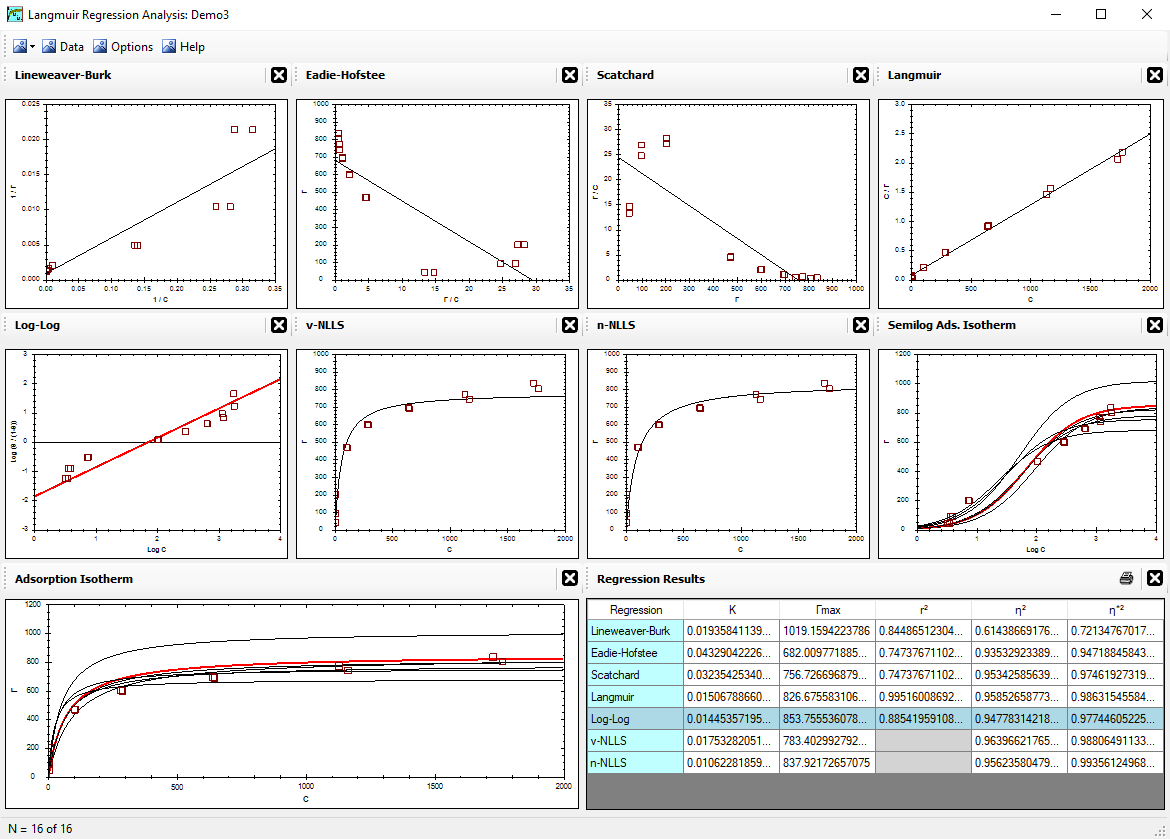Alfisol, Item 003: LMMpro, version 2.0 The Langmuir Optimization Program plus The Michaelis-Menten Optimization ProgramThe Michaelis-MentenEquation## The Michaelis-Menten Equation & Enzyme Kinetics --- an overview.

The Michaelis-Menten Equation was introduced in 1913. It correlates the initial enzyme reaction rate (v) with the amount of substrate present (S). This enzyme kinetics equation can be derived as follows:

• Let the enzyme (E) combine with the substrate to form the enzyme-substrate complex (ES), which then proceeds to form the product (P). The enzyme (E) is recycled to be used again:
 E + S k1 <=========> k-1 ES k2 <=========> k-2 E + P
where ki values are the reaction rate constants.

• The rate of production of product (P) is:
 rate = d[P]/dt = v = k2[ES] - k-2[E][P] ................. Equation 
which can be simplified since we seek to describe the initial reaction rate only. That is, the initial condition is [P] = 0. Note, that brackets [i] denote concentration of species i. Accordingly, we now have:
 v = k2[ES] ................. Equation 

• The maximum rate possible will occur when the maximum amount of enzyme exists in the ES complex form. From mass balance principles, we know that the total amount of enzyme (ET) present is the sum of the uncomplexed form (E) plus the complexed form (ES):  [E]T = [E] + [ES] ................. Equation 
Accordingly, the maximum rate (Vmax) of production of the product (P) occurs when [ES] = [E]T. Substituting this into Equation , we now have:  Vmax = k2[E]T ................. Equation 

• Similarly, the rate of production of the ES complex is:
 d[ES]/dt = k1[E][S] - k-1[ES] - k2[ES] + k-2[E][P] ................. Equation 
At the start of the process, there is no product present, [P] = 0, and this allows us to simplify Equation  when elapsed time is near zero:  d[ES]/dt = k1[E][S] - k-1[ES] - k2[ES] ................. Equation 

• It is reasonable to assume that steady state conditions are immediately established. Here, this means that the rate of production of ES is equal to the rate of decomposition of ES. Mathematically, this means d[ES]/dt = 0, where no change in concentration of ES is observed as a function of time. If we plug this into Equation  and rearrange the equation, we get:
 [ES] [E] = k1[S] k-1 + k2 = [S] KM
................. Equation 
where
 KM = k-1 + k2 k1
and KM = the Michaelis-Menten constant.

• Typically, we do not know the concentrations [E] or [ES], or, at least, these values are far too difficult to determine. So the final mathematical steps shown here seek to present an elegant expression for the reaction involved using measureable parameters. We continue with the ratio of Equations  and , and substitute with Equations  and  as needed:
 Vmax v = k2[E]T k2[ES] = [E]T [ES] = [E] + [ES] [ES] = [E] [ES] + 1 = KM [S] + 1
................. Equation 

• Rearranging Equation :
 v = Vmax [S] KM + [S]
................. Equation 
which is known as the Michaelis-Menten Equation.

If KM is known, if Vmax is known, and if the reaction is as described above, then the reaction rate (v) can be predicted for any initial substrate concentration (S). The two parameters (Vmax and KM) are obtained by regression analysis of known S and v values. It is worth noting that when S = KM, the observed reaction rate (v) will equal Vmax/2.

<Reference = CTT-3>